# SAT Math Multiple Choice Question 215: Answer and Explanation

### Test Information

Question: 215

5.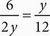Which of the following includes all possible values of y in the equation above?

• A. {-6}
• B. {-6, 6}
• C. {6}
• D. {6, 36}

B Cross-multiply to get 2y2 = 72. Solve for y to get y2 = 36, so y ± 6. Another way to solve would be to Plug In the Answers, since the question asks for a specific value and there are numbers in the answer choices. In (A), y = -6. Plug -6 into the equation and see if it works: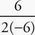=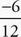, or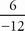=. Because this statement is true, one of the possible solutions for the equation is y = -6. Eliminate (C) and (D) because neither of these answers includes -6. According to (B), another possible value for y is 6. Plug 6 into the equation to see if it works: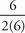=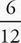, or=. Since this statement is also true, the correct answer is (B).